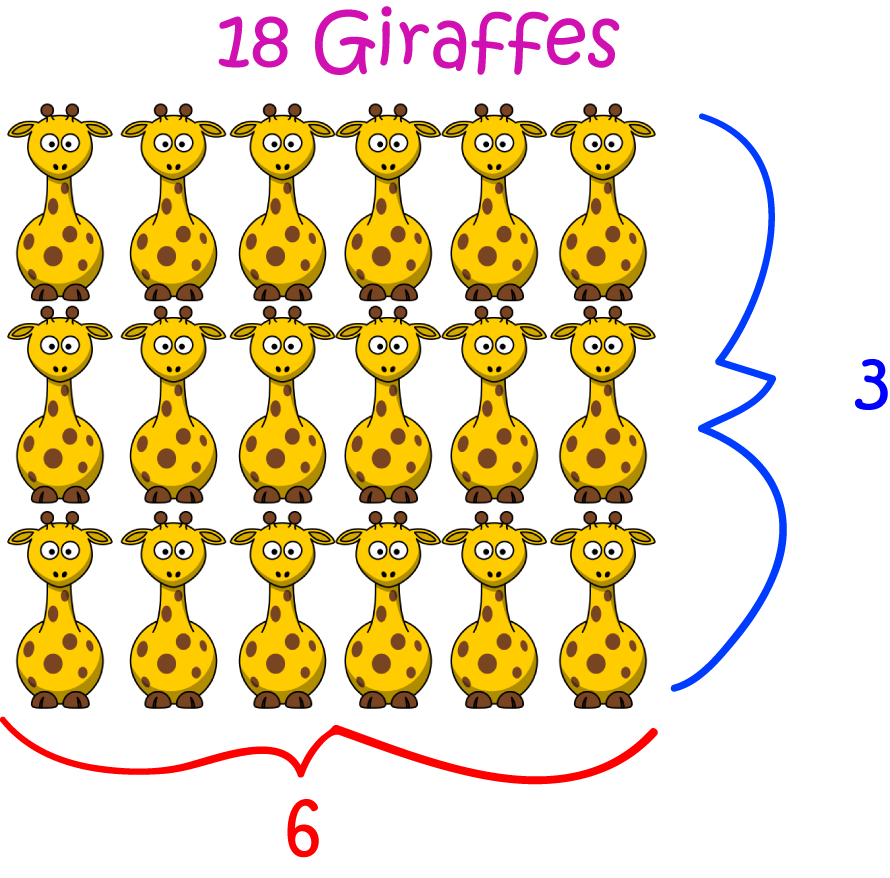# Definition of MultiplicationMultiplication is an arithmetic operation that is based on the idea of repeated addition.

For example,

$6 \times 3 = 6 + 6 + 6 = 18$.

We can multiply a number by any other numbers. So, we can multiply by fractions, decimals, negative numbers and so on.

The order of multiplication also does not matter. You get the same answer, regardless of which number you put first.

So, for example,

$4\times (-3) = (-3) \times 4 = (-3) + (-3) + (-3) + (-3) = -12.$

### Description

The aim of this dictionary is to provide definitions to common mathematical terms. Students learn a new math skill every week at school, sometimes just before they start a new skill, if they want to look at what a specific term means, this is where this dictionary will become handy and a go-to guide for a student.

### Audience

Year 1 to Year 12 students

### Learning Objectives

Learn common math terms starting with letter M

Author: Subject Coach
You must be logged in as Student to ask a Question.